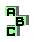NINECENTER   (4/6)

 r1619 altitude feet r1639 Jerabek hyperbola, Napoleon points r1701 orthocenter, circumcenter, orthic triangle, point X(403), homothetic triangles r1705 orthocenter, circumcenter, orthic triangle, homothetic triangles r1709 orthocenter, circumcenter, orthic triangle r1716 circumcenter, orthic triangle, isogonal points, cevian quotient r1730 isogonal points, circumconic, medial triangle r1739 circumcenter, perspector, point X(264), MacBeath conic, ninecircle, orthocentroidal circle r1749 De Longchamps point, circumcenter, altitude feet r1767 circumcenter, Jerabek hyperbola, perspective, pedal triangle r1774 circumcenter, orthic triangle r1778 circumcenter, altitude feet, isogonal points, point X(275) r1807 circumcircle, altitudes, point X(64), complement, concyclic points r1848 orthocenter r1852 ninecircle r1862 altitudes, point X(265) r1863 altitude feet, point X(52) r1864 circumcenter r1865 perspective, antimedial triangle, orthic triangle, Carnot triangle, point X(110), point X(52) r1866 orthic triangle, altitudes, circumcenter, orthocenter, similar triangles r1871 circumcenter, perspective, similar triangles r1875 incenter, Euler line, concyclic points r1876 circumcenter, complement, antipedal triangle r1880 circumcenter, orthocenter, radical center r1890 altitudes, orthic triangle1 2 3 4 5 6